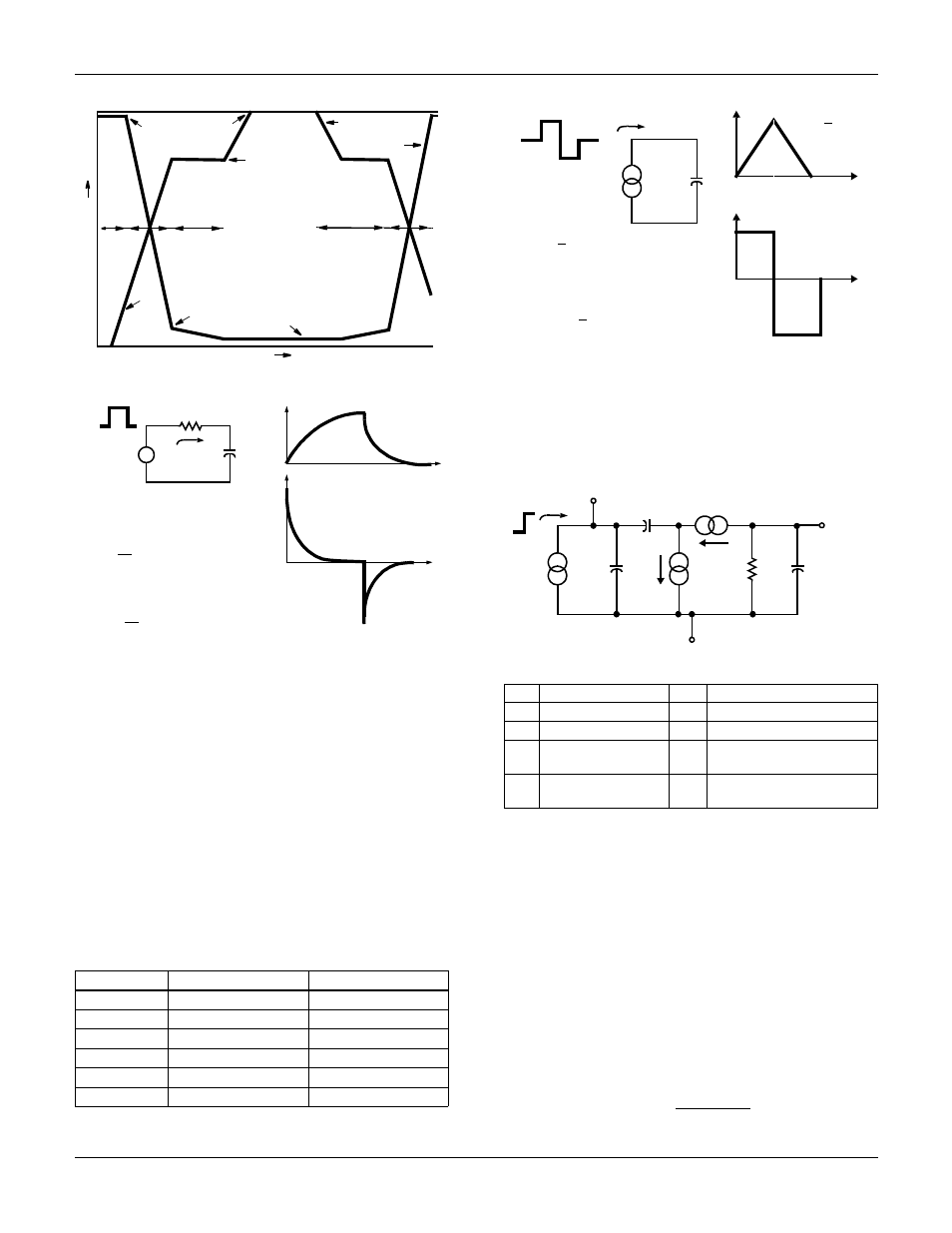# Six states, Equivalent circuit – Fairchild SEMICONDUCTOR AN-7502 User Manual

## Page 2Application Note 7502 Rev. A1

FIGURE 4.

IDEALIZED POWER MOSFET WAVEFORMS

FIGURE 5.

STEP-VOLTAGE FORCING FUNCTION

Power MOSFET devices are highly capacitive in nature;
hence, simple capacitor responses to the forcing functions
offer a good vehicle for comparison. The advantageous
choice is immediately obvious: Figure 6. Voltage/time
responses dominated by capacitance are straight lines
(when constant current is used). The slope of these lines is
proportional to current and inversely proportional to capaci-
tance. Analytically, then, constant current is most conve-
nient. It is quite another matter, however, to build a
bidirectional current drive that is accurate across the many
decades of both current and time required to establish
experimental verification.

Six States

To completely characterize power MOSFET switching wave-
forms, the six states that a device assumes, Figure 6, must

The term saturated is taken to mean a constant low-voltage drain-source

condition.

FIGURE 6.

STEP CURRENT FORCING FUNCTION

Equivalent Circuit

The lumped-parameter model of Figure 3, with the cascode-
connected JFET, can now be reduced to the linear equiva-
lent circuit of Figure 7, and the six device states investigated
from full off to full on.

FIGURE 7.

POWER MOSFET EQUIVALENT CIRCUIT

State 1: MOS Off, JFET Off

In a power-MOSFET device, no drain current will flow until
the device’s gate threshold voltage, V

gs(TH)

, is reached. Dur-

ing this time, the gate’s current drive is only charging the
gate source capacitance. More accurately, I

G

is charging

C

ISS

(C

ISS

= C

GS

+ C

GD

, C

DS

shorted), the capacitance

The current generators, g

M

V

G

and g

MJ

V

X

are open circuits

for zero drain current, and R

L

is presumed to be so low as to

represent a short circuit (generally true for practical applica-
tions). This is academic however since C

GS

is very much

larger that C

X

. The time to reach threshold, then, is simply:

STATE

MOS

JFET

Turn-on 1

Off

Off

Turn-on 2

Active

Active

Turn-on 3

Active

Saturated

Turn-off 4

Saturated

Saturated

Turn-off 5

Active

Saturated

Turn-off 6

Active

Active

1

2

3

4

5

6

STATES

I

G

= CONSTANT

GATE VOLTAGE

DRAIN

VOLTAGE

V

G(SAT)

V

D(SAT)

V

DD

V

GS

VO

L

T

AG

E

V

T

V

DK

TIME

-V

G

i(

t)

v(

t)

t

t

-I

PK

= V

G

/R

O

I

PK

= V

G

/R

O

V

G

C

v(t)

i(t)

TURN ON
v(t) = V

G

(1 - e)

i(t) = V

G

e

R

O

TURN OFF

v(t) = V

G

e

i(t) = - V

G

e

R

O

-t/R

O

C

-t/R

O

C

-t/R

O

C

-t/R

O

C

R

O

LEGEND

V

GS

- Gate Voltage

C

DS

- Drain Source Capacitance

V

X

- JFET Driving Voltage

g

M

- MOSFET Transconductance

V

D

- Drain Voltage

g

MJ

- JFET Transconductance

C

GS

- Gate Source

Capacitance

R

L

C

X

- MOSFET Feedback

Capacitance

I

G

- Constant Current Amplitude

T

1

=

C

ISS

V

gs(TH)

I

G

I

G

-I

G

i(t)

T

T

t

t

-I

G

I

G

i(

t)

v(t

)

v(t)

C

-VG =

C

I

G

T

TURN ON

v(t) =

I

G

t

C

i(t) = I

G

, 0 < t < T

TURN OFF

v(t) = 2V

G

-I

G

t

C

i(t) = I

G

, T < t < 2T

GATE

C

DS

SOURCE

DRAIN

C

X

V

GS

I

G

g

MJ

V

X

R

L

C

GS

g

M

V

G

V

D

V

X

Application Note 7502